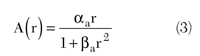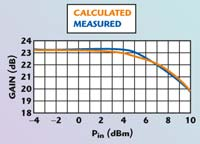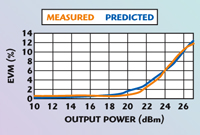www.microwavejournal.com/articles/1675-predicting-the-evm-performance-of-wlan-power-amplifiers-with-ofdm-signals

# Predicting the EVM Performance of WLAN Power Amplifiers with OFDM Signals

#### This article details a method of predicting the error vector magnitude (EVM) of wireless local area network (WLAN) power amplifiers (PA). It starts with a definition and explanation of EVM, and explains the EVM specification as defined by the IEEE 802....

May 19, 2004

The 802.11a/g WLAN standards stipulate the use of orthogonal frequency division multiplexing (OFDM) signals with M-QAM symbols modulation, where M can be as high as 64. These wideband digital modulations demand PAs with high linearity, due to the inherent high peak-to-average ratio (PAR) of these signals. This article describes the PAR and cumulative complementary distribution function (CCDF) of WLAN signals and their implications for PAs.A method is proposed to determine the PA nonlinear response, and, in turn, the EVM performance. A computer algorithm is described, which uses the proposed model to predict EVM for WLAN PAs driven with OFDM signals.

Finally, predicted versus measured EVM results are presented for two high power and high linearity WLAN power amplifiers (SKY65130 and SKY65131).Fig. 1 I-Q constellation diagram showing the error vector magnitude.WLAN IEEE 802.11a/g EVM definition

EVM is a measure of the deviation of the demodulated received symbol (I,Q) from the original transmitted data symbol (Io, Qo). The ratio of the error vector magnitude (see Figure 1) to the original symbol magnitude defines the EVM asIn practical specifications, a statistical average is taken for the EVM. The IEEE 802.11a/g standards1 define the root-mean-square EVM for an OFDM signal aswhere

Lp = length of the package (must be > symbols)
Nf = number of frames used in the EVM measurement (must be > 20)
Io, Qo = I and Q values of the original OFDM symbol
I, Q = received I and Q values
k = kth subcarrier of the OFDM symbol
Po = average power of the constellation

PA Linearity (AM/AM and AM/PM)

The linearity of a PA amplifier can be assessed from its AM/AM and AM/PM response. The AM/AM response is usually plotted as the input power versus the resulting PA gain; the AM/PM response is the input power versus the resulting phase shift introduced by the PA.

A good indication of the PA linearity is the input (or output) P1dB point. The input P1dB point is defined as the input power at which the PA gain drops by 1 dB from its small-signal gain value (see Figure 2). When the PA is operated close to its P1dB point, the output amplitude will start to be distorted (that is, it will not linearly follow changes in its input power). The same is true for the insertion phase response. Therefore, when selecting a PA for linear output power operation, it is very important to select a PA with the appropriate P1dB point.Fig. 2 Pout vs. Pin of a WLAN power amplifier showing the P1dB point.PAR for 802.11a/g OFDM Signals

The IEEE 802.11a and IEEE 802.11g standards specify the use of OFDM 64-QAM signals for increased data rates. The signal envelope of these signals is inherently very “peaky” (that is, it shows large deviation from the average power level). The variation of the input signal from its average level is described by the PAR term. The statistical distribution of the PAR is usually represented by the signal CCDF. Figure 3 shows the CCDF for an 802.11a/g input signal with the maximum specified data rate of 54 Mbps, along with a reference signal, which has a Gaussian distribution (such as AWGN).Fig. 3 CCDF of an IEEE 802.11 a/g 64-QAM OFDM signal with a data rate of 54 Mbps..A review of the CCDF summary data (left side of the figure) indicates that the signal PAR will be equal to or greater than 3.66 dB 10 percent of the time; the PAR will be equal to or greater than 6.97 dB 1 percent of the time.

Depending on the specified EVM (or bit error rate), a system may only be able to tolerate 1 percent of its total envelope being distorted, in which case the average power input to the PA should be set at least 6.97 dB below the input P1dB compression point.

For the maximum data rate of 54 Mbps, IEEE 802.11a/g specifications call for an average EVM of 5.6 percent. If the PA is backed-off by at least 5 dB from its P1dB point, the amplitude will be compressed by less than 5.6 percent.

AM/AM and AM/PM Model for WLAN PA

The EVM performance of a PA can be calculated using its AM/AM and AM/PM responses. As discussed in the previous sections the AM/AM and AM/PM responses indicate the extent to which the amplitude and phase of the input signal is distorted. If the input signal level is well below the P1dB compression point of the PA, very little AM/AM or AM/PM distortion occurs and the EVM should be very low. As the input signal approaches the 1 dB compression point of the PA, significant amplitude and phase distortion occurs and the EVM becomes larger. Therefore, to predict the EVM performance, the AM/AM and AM/PM responses of the PA must be modeled.

A PA model was developed, based on the TWT nonlinear model proposed by A. Saleh.2 The original Saleh model used the following equations to model AM/AM and AM/PM:where

r = input signal voltage levela, ßa = AM/AM response constants, typically 2 and 1, respectivelyp, ßp = AM/PM response constants, typically 2 and 3, respectively

It was found that a slight modification to Equations 3 and 4 would better model the AM/AM and AM/PM response of HBT PAs optimized for linearity and efficiency (class A/B bias). The modified equations areThe models for AM/AM and AM/PM were tested using two Skyworks WLAN power amplifiers (SKY65130 and SKY65131). These power amplifiers were built using InGaP HBT technology, and were biased for maximum linearity (high P1dB) and maximum DC PAE. Table 1 shows the main RF characteristics of these power amplifiers using 802.11g signal conditions. For these class A/B HBT power amplifiers, the model error was found to be minimum when m = 1.8 and n = 1.4.Using Equations 5 and 6, the predicted AM/AM and AM/PM responses were plotted versus the measured values, as shown in Figures 4 and 5, respectively.Fig. 4 Calculated vs. measured AM/AM response.Fig. 5 Calculated vs. measured AM/PM response.EVM Prediction Program

A Matlab program was developed to predict the EVM performance of WLAN PAs. The program generates a random bit sequence, and then maps the bits into 64-QAM symbols. The I and Q values of this sequence are stored as the Io and Qo array. The Io and Qo array is next used to form the OFDM frequency domain signal, So(f). An inverse FFT function is then used to compute the time domain signal, So(t). The input time domain signal is then scaled to the required average input power level. The resulting PA output time domain signal is calculated using Equations 5 and 6. Using the FFT function, the PA output frequency domain signal, Se(f), is calculated. From Se(f), the resulting OFDM symbols, Ie and Qe, are mapped. Equation 2 is then used to calculate the EVM of the PA. The complete program algorithm is shown in Figure 6.Fig. 6 Matlab algorithm for computing EVM from the modeled PA’s AM/AM and AM/PM responses.Predicted EVM vs. Measured EVM

The WLAN PA test samples were measured using the defined IEEE 802.11g signal under maximum data rate conditions of 54 Mbps (that is, 64-QAM, OFDM). Figure 7 (bottom right table) shows the RMS EVM which was measured at 2.5 percent for +22 dBm average output power. On the top left is the de-coded IQ constellation for 64-QAM; the bottom left shows the output spectrum mask (also shown in Figure 8, with the IEEE 802.11g specification limits).Fig. 7 WLAN PA measurements — top left: 64-QAM constellation, top right: EVM vs. carrier number, bottom left: Tx spectral mask, bottom right: RMS EVM table.Fig. 8 Output spectrum response of SKY65131 using an 802.11a/g 64-QAM, 54 Mbps input signal.To determine the accuracy of the prediction program, the EVM of the PA was measured at different levels of average input power. Figure 9 shows the predicted and measured EVM versus output power. The EVM plot shows that the predicted EVM closely matches the actual measured values. The prediction model actually has a slight over-estimation of the EVM around the inflection point of the curve. This is due to the model going into compression before the actual device. Note that the PA AM/AM response shows a slight gain expansion (~0.5 dB) about the inflection point. At this location the model is predicting a higher level of gain compression.Fig. 9 Predicted vs. measured EVM for a WLAN PA.Conclusion

This article describes the EVM requirements for WLAN OFDM applications and the importance of PA linearity in order to achieve adequate EVM, along with a review of the 1 dB compression point and the CCDF and PAR characteristics of WLAN OFDM signals. It further proposes a method for predicting the amplitude and phase distortion introduced by a WLAN PA when driven into its nonlinear region of operation. A computer algorithm is described that uses the proposed PA nonlinear model to predict the EVM performance.

The EVM model was compared to the measurements taken from sample WLAN power amplifiers using a 64-QAM OFDM signal with a maximum data rate of 54 Mbps. The results show the model very closely predicts the measured performance of the power amplifiers.

References

1. IEEE Std. 802.11a–1999, Part 11: Wireless LAN Medium Access Control (MAC) and Physical Layer (PHY) Specifications.
2. A. Saleh, “Frequency-independent and Frequency-dependent Nonlinear Models of TWT Amplifiers,” IEEE Transactions on Communications, Vol. 29, No. 11, November 1981.

Patrick Naraine earned his BSEE and MSEE degrees from McMaster University, Hamilton, Ontario, Canada. He was technical payload manager of Canada’s first remote sensing satellite built for the Canadian Space Agency in 1996. He then joined Nortel Networks, where he was a group leader in charge of RF system designs for AMPS, TDMA and EDGE base station transceivers. From 1999 to 2002, he worked at AT&T Wireless on the system design, testing and deployment of the first US commercial fixed wireless voice and high speed Internet system using OFDM modulated signals. He is currently working on system designs for RFICs, MMICs and PAMs at Skyworks Solutions Inc. He can be reached via e-mail at patrick.naraine@skyworksinc.com.